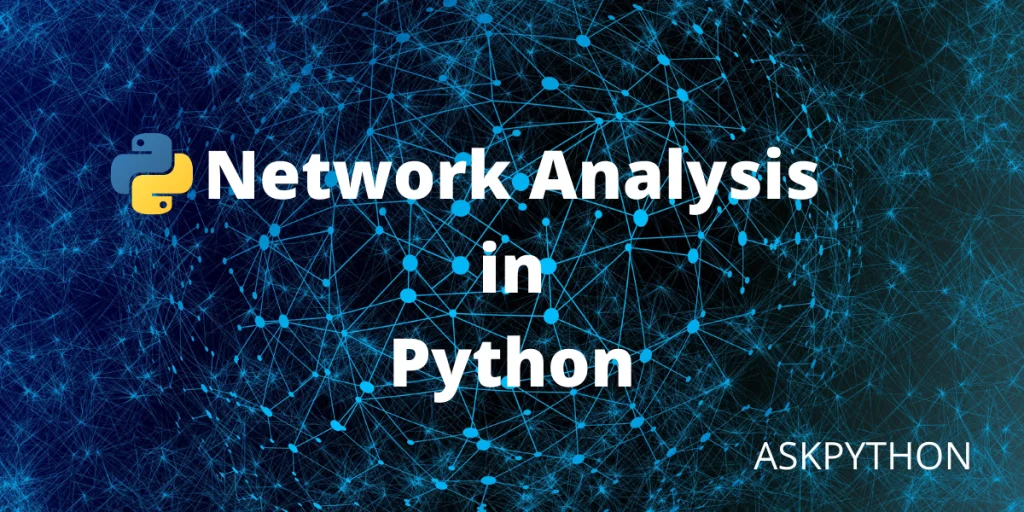# Network Analysis in Python – A Complete GuideAn approach for evaluating, managing, and tracking processes of management and workflows are called network analysis. Moreover, data analysis helps in creating graphical diagrams of nodes and elements of the structure, but unlike a workflow, a network diagram examines the chronological series of events, objectives, and assignments, along with their timeframes and dependencies, and depicts them visually as a tree or as a table, such as in a Gantt chart.

When developing a project plan, project leaders might need network analysis as it helps in dealing with the following factors:

• Inter – dependence of tasks
• The duration between actions and how they should be effectively buffered.
• Start and end dates, first from earliest to one of the most current
• Activity intervals
• Developing the path for most important tasks and activities.

The network analysis method is commonly used within the design to the development phase, to enhance project control and make sure tasks are delivered on time and within budget.

## How to implement network analysis in Python

There are many ways of doing network analysis in Python. Moreover, many tools are available to plot network analysis graphs, but in this article, we will be specifically using networkx and matplotlib as these are powerful network plotting tools.

We will be understanding network plotting by using some user databases available online. In this example, we have fetched two 16th century based Chinese population records, that may have lived at the time the author of a famous novel lived, and we will try to create a graph of people that might have known him.

### Let’s start by importing packages

```import networkx as nx
import matplotlib.pyplot as plt
```

There are multiple files in the git folder, but we will be needing only, ‘edges.tsv’ and ‘nodes.tsv’. These word files contain all the historical data.

These historical databases are in .tsv file format. As you see in the above image, the data is scattered and unfiltered. To graph this data, we need to segregate it, so that the compiler can start reading the data easily.

The code below demonstrates how to load these files into a (get_data) method and segregate them as per our need.

```def data_extraction(name_ofile):
with open(name_ofile, 'r', encoding='utf8') as rf:
# transform file into string and split along new line

# new line will be created at tab spaces
filedata = [line.split("\t") for line in filelines]

filedata = filedata[1:]

# load data in from file
```

### Creating the graph and adding node information toit:

```Graph = nxnas.Graph()

# graph gets data of node added to it
for nxnode in data_ofnode:
# sequentially adding id, name, chinese name, and index year

#  graph gets data of edge added to it
for nxedge in data_ofedge:
# sequentially adding node 1, node 2, kin, and label

```

### Adding data metrics for the graph

```degree_centrality = nxnas.degree_centrality(Graph)
closeness_centrality = nxnas.closeness_centrality(Graph)
betweenness_centrality = nxnas.betweenness_centrality(Graph)
```

Metrics are a wide variety of algorithms that are present in the networkx python package that lets you study your network. In this example, we’ve used three data metrics to plot our graph. Let’s understand their functions and purpose.

• Degree centrality: The number of edges a node has.
• Closeness_centrality: Finds the nodes with the slightest distance between them. Through this way the efficiecny of nodes to transfer data is measured.
• Betweeness centrality: Finds shortest path.

### Complete Code for Network Analysis in Python

```import networkx as nxnas
import matplotlib.pyplot as myplot

# This function is employed to extract data from the .tsv files
def data_extraction(name_ofile):
# Here, a data loading will be done through a context manager
with open(name_ofile, 'r', encoding='utf8') as rf:
# transform file into string and split along new line

# new line will be created at tab spaces
filedata = [line.split("\t") for line in filelines]

filedata = filedata[1:]

# load data in from file

# create graph object
Graph = nxnas.Graph()

# graph gets data of node added to it
for nxnode in data_ofnode:
# sequentially adding id, name, chinese name, and index year

#  graph gets data of edge added to it
for nxedge in data_ofedge:
# sequentially adding node 1, node 2, kin, and label

# Data metrics for the graph
degree_centrality = nxnas.degree_centrality(Graph)
closeness_centrality = nxnas.closeness_centrality(Graph)
betweenness_centrality = nxnas.betweenness_centrality(Graph)

# The process of depicting the graph
nxnas.draw_spring(Graph)
myplot.show()
```